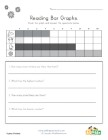This group of free graphing worksheets is a good introduction for kids learning to read graphs. Kids are asked to look at the graph in each worksheet and then to use it to answer the simple questions. If your children can do these worksheets, they will have gotten and good start on learning about graphing and bar charts.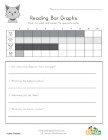Animal Themed Bar Graph Worksheet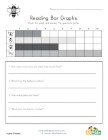Bug Themed Bar Graph Worksheet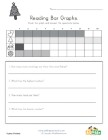Christmas Bar Graph Worksheet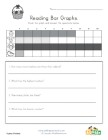Easter Bar Graph Worksheet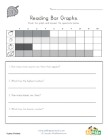Fall Bar Graph Worksheet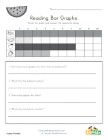Fruit Themed Bar Graph Worksheet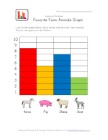Graphing Worksheet with Animals Theme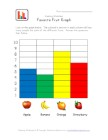Graphing Worksheet with Fruit Theme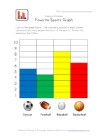Graphing Worksheet with Sports Theme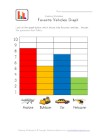Graphing Worksheet with Vehicles Theme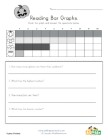Halloween Bar Graph Worksheet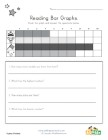Memorial Day Bar Graph Worksheet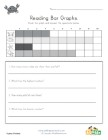Ocean Themed Bar Graph Worksheet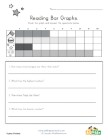Patriotic Bar Graph Worksheet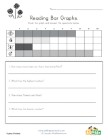Spring Bar Graph Worksheet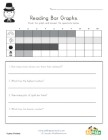St. Patrick's Day Bar Graph Worksheet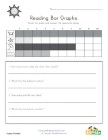Summer Bar Graph Worksheet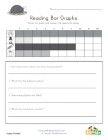Thanksgiving Bar Graph Worksheet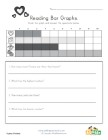Valentine's Day Bar Graph Worksheet